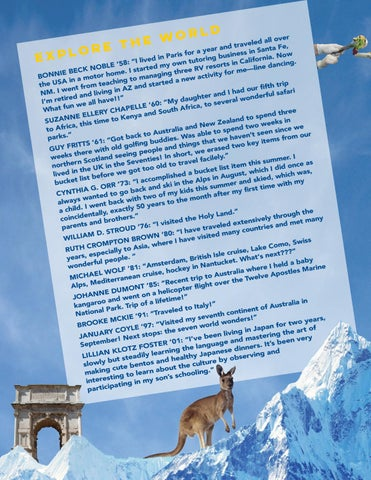rld o over w led all e e v a h r t d Fe, e t year an ess in Santa r a r o o f Now Paris pl busin

ex

in nia. ing “I lived Califor n tutor : in 8 w ing. 5 o s ’ t r y E o dm e danc res OBL e n t li V N r R a — K t e e s C e. I thre for m IE BE tor hom to managing new activity BONN o m a A in ted a aching trip the US nd star rom te f a t Z n ur fifth A e o w in d I a g . h fari in I NM and liv erful sa er and t d d h n e ” g o ir ! u t ! w a e e I’m r veral “My d all hav E ’60: a, to se L un we ic f L r f t E a A P h W CHA outh ree a and S end th ELLERY y p E n s e N o K t N o in land SUZA time t ew Zea d two weeks N a, this ic d r n f a A n e we lia to to spe en sinc Austra e le s b o t t a ’ ” . k s n our s c a e park Got ba dies. W s that we hav ey items from “ d : u 1 b 6 ’ g wo k RITTS thing golfin GUY F rased t le and ith old e p w o e e e w r p , e th short eeing r. I weeks ilely.” ies! In tland s t o n c e S summe ce as v vel fac n e a is r r S h e t t e h o t h t r t m no in old id on st ite the UK ot too hich I d ucket li w as, b , a t s d u lived in t before we g hich w ug w lishe A , p in d s m s li ie o k c t my he Alp and s : “I ac bucke mmer ski in t e with RR ’73 u d s O im n t . is a t G h s k t r IA y fi kids o bac CYNTH after m of my ed to g h o t t n w n t a o h w m ck wit o the always ent ba years t w 0 I 5 . d ly il a ch exact h the and.” ntally, e id c throug rs.” Holy L e in ly y e h e h t co t o iv r s d ite db et man ten n is x m a v e d I s d t “ n n a : le pare tries D ’76 trave y coun STROU n “I have . a : D 0 m 8 ’ d M e visite ROWN WILLIA s e I hav TON B r P e h o, Swis M w m O , o R C ia C s e RUTH y to A e, Lak ???” speciall le cruis e s I , s h r “ ‘s next is a t . it a le r ye h p B o , W ket. baby erdam rful pe Nantuc I held a “Amst e wonde : in e 1 r y 8 e ’ e h k w oc s Marin a h OLF li le , t a W s e r t o L is s p u E to Au lve A ean cr MICHA nt trip he Twe iterran t e c d r e e e R v M “ o , Alps T ’85: flight UMON copter li D e E h N a JOHAN and went on e!” lifetim oo a r a f alia in o g n a ip k Italy!” . Tr f Austr k o o r t a t n P d e l le a contin “Trave Nation eventh IE ’91: s !” K y C m M years, ed onders KE it w is O V O ld r two “ r R o : o f B 7 w 9 n ’ a n of YLE seve in Jap RY CO the art ps: the living g o n t n i s e r t e e JANUA t x b s e very “I’ve d ma ber! N R ’01: ’s been age an t E u I T Septem g . S s n r a O l e the TZ F dinn nd N KLO arning anese e p l a y J l i y rving a d LILLIA h e a t s l e b a t s e o but and h re by slowly e cultu bentos h t e t t u u c o g.” making g to learn ab choolin s n ’s i t n s o my s intere ting in a p i c i t par

school magazine spring

2019

8